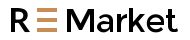Free Shipping on orders over US\$39.99

# How to Generate Tiny DC Supply Voltages of Hundreds of mV

For many years, linear and switching regulators have used feedback voltages of about 1.2 V. This voltage is generated by a bandgap circuit in the DC-DC converter IC, which determines the lowest voltage that can be set using an external resistor divider. By now, most modern regulator ICs can generate output voltages of 0.8 V, 0.6 V, or even 0.5 V. The internal reference is also designed in this way, so lower voltages can be obtained. Figure 1 shows this type of switching regulator, the LTC3822, which generates a 0.6 V feedback voltage from a 0.6 V reference voltage.

What are some good solutions to generate tiny DC supply voltages of only a few hundred millivolts?
Answer: Simply connect a clean, applied positive voltage to the feedback resistor of the DC-DC converter.

Over the past few years, the supply voltage of Electronic components has continued to drop due to the shrinking geometry of digital circuits such as microcontrollers, CPUs, DSPs, etc. There are also applications in the measurement field that require low supply voltages.

For many years, linear and switching regulators have used feedback voltages of about 1.2 V. This voltage is generated by a bandgap circuit in the DC-DC converter IC, which determines the lowest voltage that can be set using an external resistor divider. By now, most modern regulator ICs can generate output voltages of 0.8 V, 0.6 V, or even 0.5 V. The internal reference is also designed in this way, so lower voltages can be obtained. Figure 1 shows this type of switching regulator, the LTC3822, which generates a 0.6 V feedback voltage from a 0.6 V reference voltage.Figure 1. LTC3822 DC-DC Converter Produces Low Output Voltages of 0.6 V or More

However, if a supply voltage lower than 0.6 V is required, the circuit shown in Figure 1 needs to be adjusted, otherwise it cannot be used.

With a few tricks, you can also make a switching or linear regulator produce a voltage lower than the feedback voltage. This can be achieved by using the circuit shown in Figure 2. Connect the resistive divider with an external bias positive voltage to regulate the output voltage. This voltage can be generated by a low dropout regulator (LDO) or a voltage reference. In this way, the resistor divider forms a voltage divider, and the current IFB flows in the opposite direction to the normal case in Figure 1. In Figure 2, current flows from an external reference to the output voltage through a resistive divider.

Equation 1 shows the relationship between the IC’s feedback voltage (VFB), the desired output voltage (VOUT), the applied positive DC bias voltage (VOFFSET), and the resistor divider resistors R1 and R2.For resistor divider value selection, it is recommended that the sum of R1, R2 be between 100 kΩ and 500 kΩ. This keeps the bias current low enough in terms of power efficiency, but high enough to prevent excessive noise coupling into the sensitive feedback path.Figure 2. Adjusting the circuit of Figure 1 to produce output voltages below 0.6 V

This design philosophy is typically used to generate voltages that are lower than the minimum voltage rating of a switching regulator or linear regulator. However, a few points should be noted: the applied voltage reference should be up and running before the DC-DC converter is turned on. If this auxiliary voltage is 0 V or has high resistance, the DC-DC converter may generate excessive voltage and damage the load circuit.

In the worst case, when the switching regulator has not turned on but the auxiliary voltage has been applied, the current IFB flowing through the resistor divider will charge the output capacitor to a voltage higher than the set voltage. This happens when the load has extremely high impedance. So setting a minimum load to avoid this may be necessary.

The accuracy of the resistor divider’s auxiliary voltage (1 V in Figure 2) directly affects the resulting supply voltage accuracy. Therefore, a particularly clean low ripple voltage should be used.

Also, not all voltage converters are suitable for this type of operation. For example, the measurement range of a current-sense amplifier in a DC-DC converter may only provide an operating range at higher voltages. It should also be noted that producing very low voltages at higher input voltages also requires a low duty cycle. Here, it can be very helpful to choose a switching regulator IC that has a short minimum on-time and operates at a low switching frequency.Figure 3. Initial testing of the circuit can be performed using a simulation tool such as ADI’s LTspice®

Using a simulation tool such as ADI’s LTspice is useful for an initial check if you want to run a linear or switching regulator with an output voltage lower than the IC manufacturer’s specified output voltage. Figure 3 shows a circuit built with the LTC3822 using an additional voltage source as a bias for the feedback path. In this circuit, an output voltage of 200 mV is produced. According to the data sheet, the LTC3822 is suitable for generating output voltages down to 0.6 V. In the circuit, the auxiliary voltage source (voltage source V2 in Figure 3) can be implemented by an LDO regulator or a voltage reference. Using the techniques described in this article, fully test the circuit and possibly produce even lower output voltages.